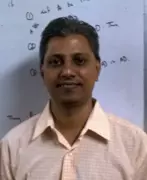X
On certain positivity classes of operators
Published in Taylor and Francis Inc.
2016
Volume: 37

Issue: 2
Pages: 206 - 224
Abstract
A real square matrix A is called a P-matrix if all its principal minors are positive. Such a matrix can be characterized by the sign non-reversal property. Taking a cue from this, the notion of a P-operator is extended to infinite dimensional spaces as the first objective. Relationships between invertibility of some subsets of intervals of operators and certain P-operators are then established. These generalize the corresponding results in the matrix case. The inheritance of the property of a P-operator by the Schur complement and the principal pivot transform is also proved. If A is an invertible M-matrix, then there is a positive vector whose image under A is also positive. As the second goal, this and another result on intervals of M-matrices are generalized to operators over Banach spaces. Towards the third objective, the concept of a Q-operator is proposed, generalizing the well known Q-matrix property. An important result, which establishes connections between Q-operators and invertible M-operators, is proved for Hilbert space operators. © 2016 Taylor & Francis Group, LLC.
Authors (1)
•Concepts (11)
•Banach spaces
•Vector spaces
•Infinite dimensional
•INVERTIBILITY
•MATRIX CASE
•PRINCIPAL MINOR
•PRINCIPAL PIVOT TRANSFORMS
•Schur complement
•SPACE OPERATORS
•Square matrices
•Matrix algebra